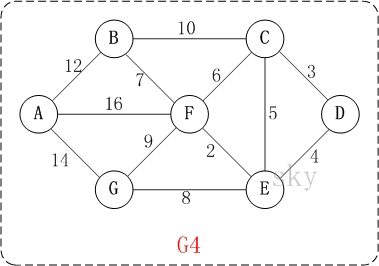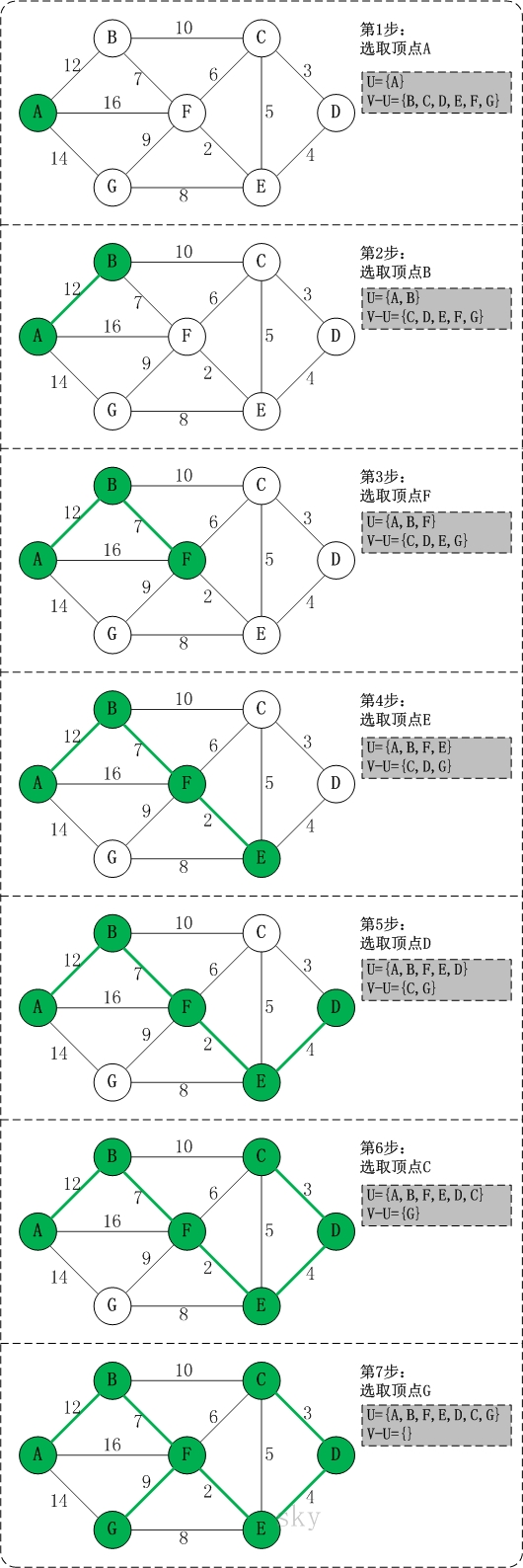二、普里姆算法图解​ 此时，U={A}。

​ 上一步操作之后，U={A}, V-U={B,C,D,E,F,G}；因此，边(A,B)的权值最小。将顶点B添加到U中；此时，U={A,B}。

​ 上一步操作之后，U={A,B}, V-U={C,D,E,F,G}；因此，边(B,F)的权值最小。将顶点F添加到U中；此时，U={A,B,F}。

​ 上一步操作之后，U={A,B,F}, V-U={C,D,E,G}；因此，边(F,E)的权值最小。将顶点E添加到U中；此时，U={A,B,F,E}。

​ 上一步操作之后，U={A,B,F,E}, V-U={C,D,G}；因此，边(E,D)的权值最小。将顶点D添加到U中；此时，U={A,B,F,E,D}。

​ 上一步操作之后，U={A,B,F,E,D}, V-U={C,G}；因此，边(D,C)的权值最小。将顶点C添加到U中；此时，U={A,B,F,E,D,C}。

​ 上一步操作之后，U={A,B,F,E,D,C}, V-U={G}；因此，边(F,G)的权值最小。将顶点G添加到U中；此时，U=V。

三、普里姆算法的代码说明

1. 基本定义

public class MatrixUDG {

private char[] mVexs;       // 顶点集合
private int[][] mMatrix;    // 邻接矩阵
private static final int INF = Integer.MAX_VALUE;   // 最大值

...
}

MatrixUDG是邻接矩阵对应的结构体。mVexs用于保存顶点，mEdgNum用于保存边数，mMatrix则是用于保存矩阵信息的二维数组。例如，mMatrix[i][j]=1，则表示"顶点i(即mVexs[i])"和"顶点j(即mVexs[j])"是邻接点；mMatrix[i][j]=0，则表示它们不是邻接点。

2. 普里姆算法

/*
* prim最小生成树
*
* 参数说明：
*   start -- 从图中的第start个元素开始，生成最小树
*/
public void prim(int start) {
int num = mVexs.length;         // 顶点个数
int index=0;                    // prim最小树的索引，即prims数组的索引
char[] prims  = new char[num];  // prim最小树的结果数组
int[] weights = new int[num];   // 顶点间边的权值

// prim最小生成树中第一个数是"图中第start个顶点"，因为是从start开始的。
prims[index++] = mVexs[start];

// 初始化"顶点的权值数组"，
// 将每个顶点的权值初始化为"第start个顶点"到"该顶点"的权值。
for (int i = 0; i < num; i++ )
weights[i] = mMatrix[start][i];
// 将第start个顶点的权值初始化为0。
// 可以理解为"第start个顶点到它自身的距离为0"。
weights[start] = 0;

for (int i = 0; i < num; i++) {
// 由于从start开始的，因此不需要再对第start个顶点进行处理。
if(start == i)
continue;

int j = 0;
int k = 0;
int min = INF;
// 在未被加入到最小生成树的顶点中，找出权值最小的顶点。
while (j < num) {
// 若weights[j]=0，意味着"第j个节点已经被排序过"(或者说已经加入了最小生成树中)。
if (weights[j] != 0 && weights[j] < min) {
min = weights[j];
k = j;
}
j++;
}

// 经过上面的处理后，在未被加入到最小生成树的顶点中，权值最小的顶点是第k个顶点。
// 将第k个顶点加入到最小生成树的结果数组中
prims[index++] = mVexs[k];
// 将"第k个顶点的权值"标记为0，意味着第k个顶点已经排序过了(或者说已经加入了最小树结果中)。
weights[k] = 0;
// 当第k个顶点被加入到最小生成树的结果数组中之后，更新其它顶点的权值。
for (j = 0 ; j < num; j++) {
// 当第j个节点没有被处理，并且需要更新时才被更新。
if (weights[j] != 0 && mMatrix[k][j] < weights[j])
weights[j] = mMatrix[k][j];
}
}

// 计算最小生成树的权值
int sum = 0;
for (int i = 1; i < index; i++) {
int min = INF;
// 获取prims[i]在mMatrix中的位置
int n = getPosition(prims[i]);
// 在vexs[0...i]中，找出到j的权值最小的顶点。
for (int j = 0; j < i; j++) {
int m = getPosition(prims[j]);
if (mMatrix[m][n]<min)
min = mMatrix[m][n];
}
sum += min;
}
// 打印最小生成树
System.out.printf("PRIM(%c)=%d: ", mVexs[start], sum);
for (int i = 0; i < index; i++)
System.out.printf("%c ", prims[i]);
System.out.printf("\n");
}